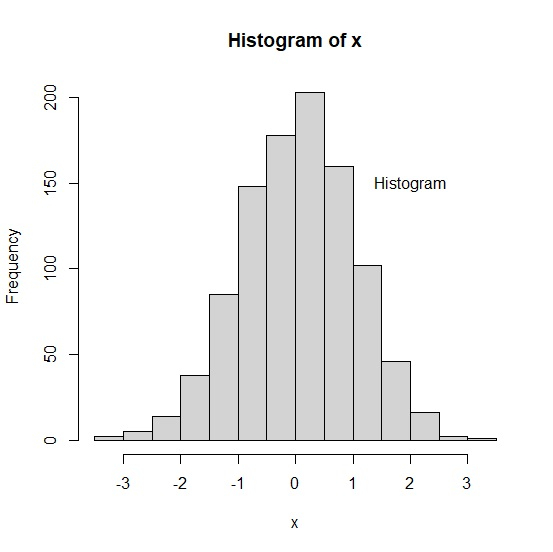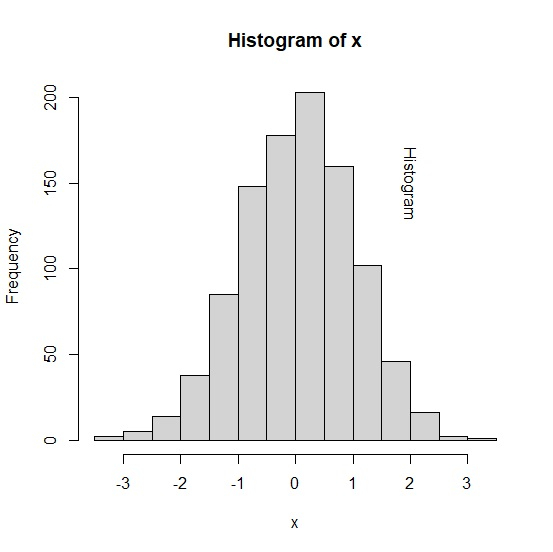# How to display text in base R plot with 180 degrees rotation?

To display text in base R plot with 180 degrees rotation, we can use text function. We would need to define both the axes values for the position where we need the text to be rotated inside the plot. For the rotation of the text, srt argument will be used and set to the value equals to 270. Check out the below example to understand how it works.

## Example

x<-rnorm(1000)
hist(x)
text(x=2,y=150,"Histogram")

## Output## Example

hist(x)
text(x=2,y=150,"Histogram",srt=270)

## Output